## 1、题目

``````
package pers.mobian.questions01;

public class test01 {
public static void main(String[] args) {
int i = 1;
i = i++;
int j = i++;
int k = i + ++i * i++;
System.out.println("i="+i);
System.out.println("j="+j);
System.out.println("k="+k);
}
}
``````

## 2、分析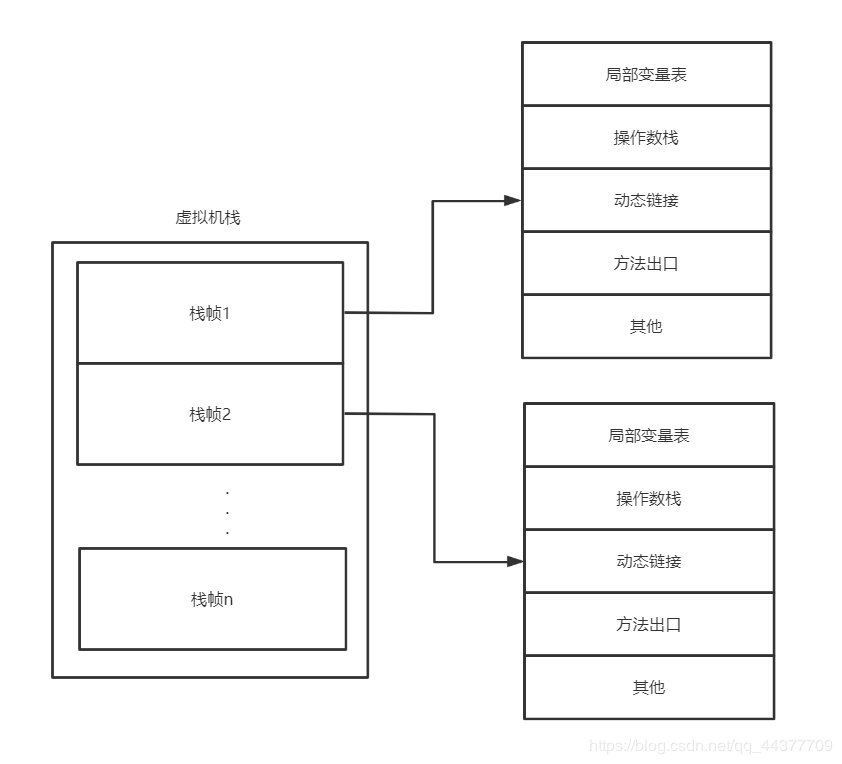### 2.1、第一步

int i = 1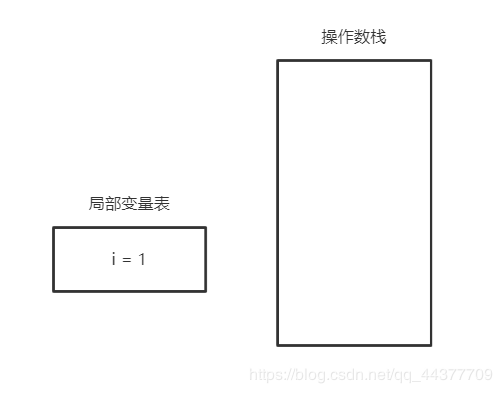### 2.2、第二步

i = i++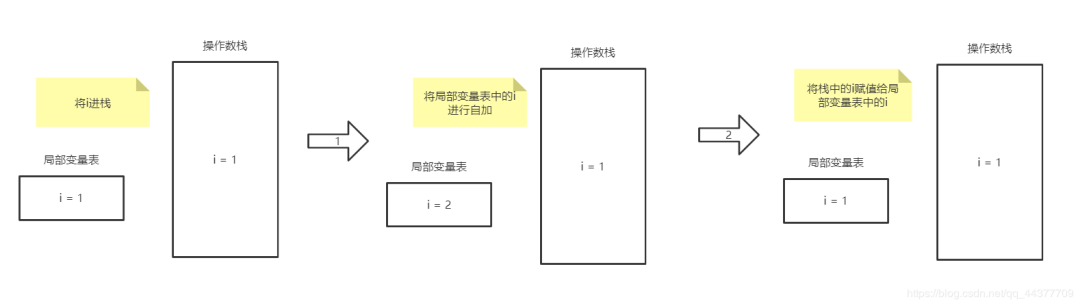### 2.3、第三步

int j = i++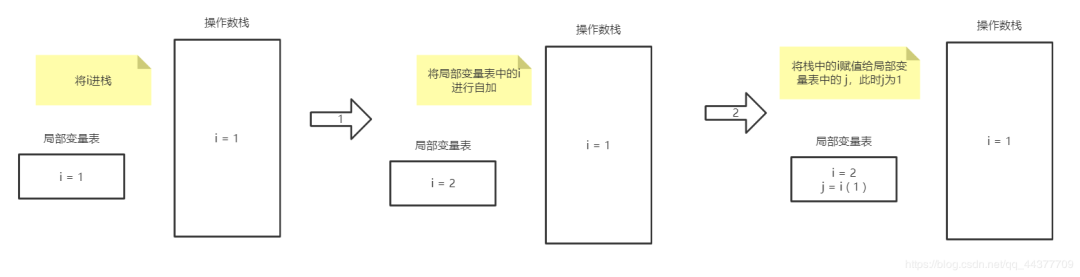### 2.4、第四步

int k = i + ++i * i++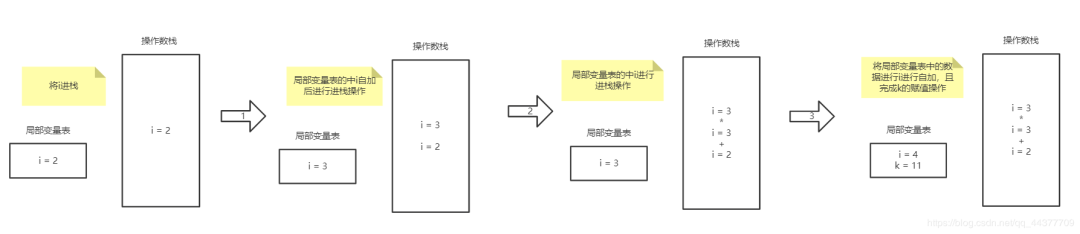### 2.5、结果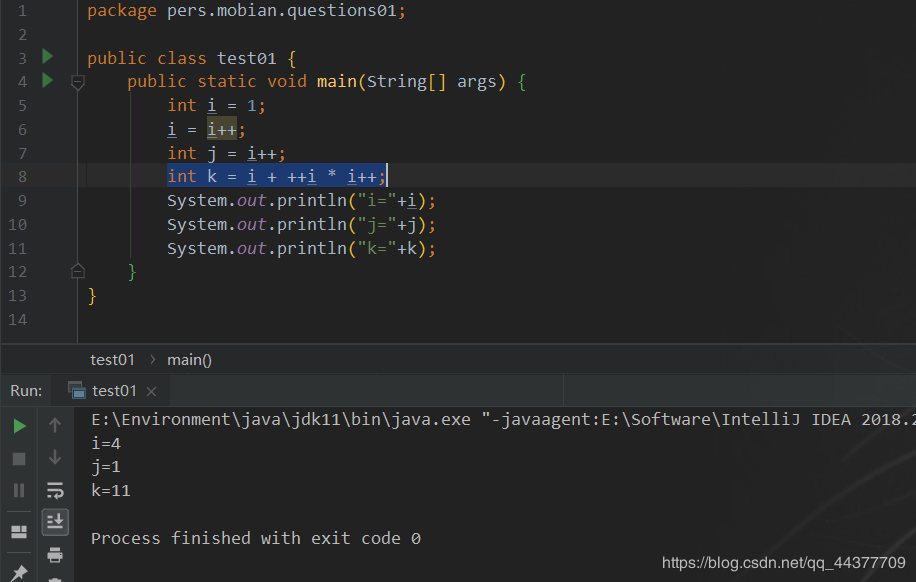## 3、i = ++i

``````
public class test02 {
public static void main(String[] args) {
int i = 1;
i = ++i;
System.out.println(i); // 结果：i = 2
}
}
``````

``````

2. 后台回复「Java面试」即可获取

``````

上一篇直观讲解一下 RPC 调用和 HTTP 调用的区别！

2021-04-04深入认识二进制序列化--记一次生产事故的思考

2021-04-04